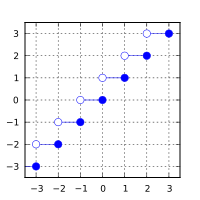/C

# ceil, ceilf, ceill

Defined in header `<math.h>`
`float       ceilf( float arg );`
(1) (since C99)
`double      ceil( double arg );`
(2)
`long double ceill( long double arg );`
(3) (since C99)
Defined in header `<tgmath.h>`
`#define ceil( arg )`
(4) (since C99)
1-3) Computes the smallest integer value not less than `arg`.
4) Type-generic macro: If `arg` has type `long double`, `ceill` is called. Otherwise, if `arg` has integer type or the type `double`, `ceil` is called. Otherwise, `ceilf` is called.

### Parameters

 arg - floating point value

### Return value

If no errors occur, the smallest integer value not less than `arg`, that is ⌈arg⌉, is returned.

Return valueArgument

### Error handling

Errors are reported as specified in math_errhandling.

If the implementation supports IEEE floating-point arithmetic (IEC 60559),

• The current rounding mode has no effect.
• If `arg` is ±∞, it is returned, unmodified
• If `arg` is ±0, it is returned, unmodified
• If arg is NaN, NaN is returned

`FE_INEXACT` may be (but isn't required to be) raised when rounding a non-integer finite value.

The largest representable floating-point values are exact integers in all standard floating-point formats, so this function never overflows on its own; however the result may overflow any integer type (including `intmax_t`), when stored in an integer variable.

This function (for double argument) behaves as if (except for the freedom to not raise `FE_INEXACT`) implemented by.

```#include <math.h>
#include <fenv.h>
#pragma STDC FENV_ACCESS ON
double ceil(double x)
{
double result;
int save_round = fegetround();
fesetround(FE_UPWARD);
result = rint(x); // or nearbyint
fesetround(save_round);
return result;
}```

### Example

```#include <math.h>
#include <stdio.h>
int main(void)
{
printf("ceil(+2.4) = %+.1f\n", ceil(2.4));
printf("ceil(-2.4) = %+.1f\n", ceil(-2.4));
printf("ceil(-0.0) = %+.1f\n", ceil(-0.0));
printf("ceil(-Inf) = %+f\n",   ceil(-INFINITY));
}```

Possible output:

```ceil(+2.4) = +3.0
ceil(-2.4) = -2.0
ceil(-0.0) = -0.0
ceil(-Inf) = -inf```
• C11 standard (ISO/IEC 9899:2011):
• 7.12.9.1 The ceil functions (p: 251)
• 7.25 Type-generic math <tgmath.h> (p: 373-375)
• F.10.6.1 The ceil functions (p: 526)
• C99 standard (ISO/IEC 9899:1999):
• 7.12.9.1 The ceil functions (p: 231-232)
• 7.22 Type-generic math <tgmath.h> (p: 335-337)
• F.9.6.1 The ceil functions (p: 462-463)
• C89/C90 standard (ISO/IEC 9899:1990):
• 4.5.6.1 The ceil function

### See also

 floorfloorffloorl (C99)(C99) computes largest integer not greater than the given value (function) trunctruncftruncl (C99)(C99)(C99) rounds to nearest integer not greater in magnitude than the given value (function) roundlroundllround (C99)(C99)(C99) rounds to nearest integer, rounding away from zero in halfway cases (function) nearbyintnearbyintfnearbyintl (C99)(C99)(C99) rounds to an integer using current rounding mode (function) rintrintfrintllrintlrintflrintlllrintllrintfllrintl (C99)(C99)(C99)(C99)(C99)(C99)(C99)(C99)(C99) rounds to an integer using current rounding mode with exception if the result differs (function)

© cppreference.com
Licensed under the Creative Commons Attribution-ShareAlike Unported License v3.0.
http://en.cppreference.com/w/c/numeric/math/ceil